Maths-
General
Easy

Question

# The cartesian equation of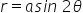is

##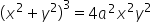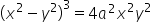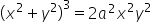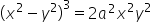Hint:

## The correct answer is:### Using the formula, we may generate an endless number of polar coordinates for a single coordinate point.(r, +2n) or (-r, +(2n+1)) are possible formulas, where n is an integer.If measured in the opposite direction, the value of is positive.When calculated counterclockwise, the value of is negative.If laid off at the terminal side of, the value of r is positive.If r is terminated at the prolongation through the origin from the terminal side of, it has a negative value.The equation is given asHere we used the concept of the polar coordinate system and also the trigonometric ratios to find the solution. So the equation is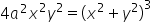.#### With Turito Foundation.#### Get an Expert Advice From Turito.